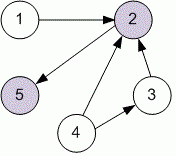Competitions

# Cow Contest

Time limit 1 second
Memory limit 128 MiB

n cows, conveniently numbered 1..n, are participating in a programming contest. As we all know, some cows code better than others. Each cow has a certain constant skill rating that is unique among the competitors.

The contest is conducted in several head-to-head rounds, each between two cows. If cow a has a greater skill level than cow b (1a, bn, ab), then cow a will always beat cow b.

Farmer John is trying to rank the cows by skill level. Given a list the results of m two-cow rounds, determine the number of cows whose ranks can be precisely determined from the results. It is guaranteed that the results of the rounds will not be contradictory.

## Input data

The first line contains two integers n (1n100) and m (1m4500). Each of the next m lines contains two integers that describe the competitors and results (the first integer a, is the winner) of a single round of competition: a and b.

## Output data

Print a single integer representing the number of cows whose ranks can be determined.## Examples

Input example #1
5 5
4 3
4 2
3 2
1 2
2 5

Output example #1
2

Source 2008 USACO January Silver